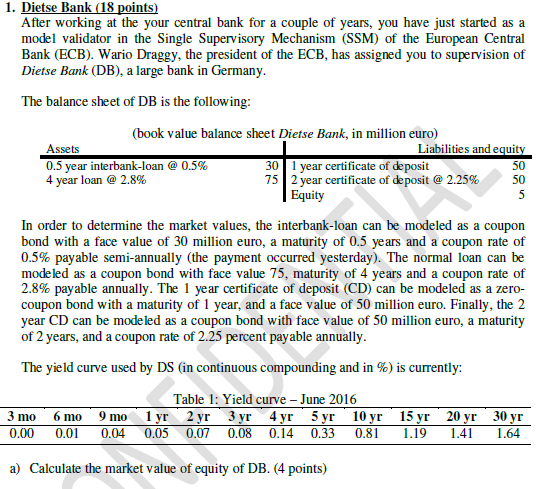# (partial) Duration and convexity

Hello everyone,
Currently Im studying for my banking, insurance and risk exam and need some help with two questions of a test exam im making.
The exam has its answer already displayed. However, I cant get to the exact calculations and answer of question b and c.
At question b I am able to compute everything right, except for the duration and convexity of 0,86.
At question c I am unable to get the exact right answers on all 3 questions. I get very close (like 3,84 at partial duration and 1,98 at liabilities).
Could anyone help me out? I would be very gratefull.Market value of assets:
0,5 year loan 30,073
4 year loan 82,96

market value of liabilities
1 year CD 49,98
2 year CD
52,178

market value of equity 10,88

Question B
Calculate the duration and convexity of assets and liabilities, and use both to predict the market value of equity would be when the yield curve shifts upwards in a parallel fashion with 7%. how would your answer change when you only use duration and diregard convexity?

0,5 year interbank Duration=0,5, convexity =0,25
4 year loan Duration =3,848, convexity = 15,14
1 year CD Duration =1 convexity =1
2 year CD Duration =1,978, convexity = 3,935

Duration only:
New equity = 10,88-12,67=-1,79

Duration and convexity:
New equity = 10,88 - 10,02 = 0.86 (this is the one I am unable to answer in a correct way)

Question C
Use the partial duration gap approach to calculate the market value of equity when the zero-rates up starting from (and including) 2 years are increased by 1,5 percentage points)

Partial duration of assets (and including) 2 years = 3,823
Partial duration of liabilities (and including) 2 years = 1,957

New equity = 10,88-3,22=7,66

Kind regards,
Luc